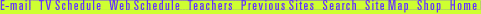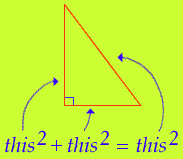## Pythagorean Puzzle

Here's the deal; there was this Greek guy named Pythagoras, who lived over 2,000 years ago during the sixth century B.C.E. Pythagoras spent a lot of time thinking about math, astronomy, and music. One idea he came up with was a mathematical equation that's used all the time, for example in architecture, construction, and measurement.

His equation is simple: a2 + b2 = c2.

What it means is that in a right triangle (where one angle equals 90°), the sum of the squares of two sides equals the square of the hypotenuse (the longest side).

Or, to put it another way:Check it out—you can show that the Pythagorean theorem works.

Andrew Wiles | Math's Hidden Woman | Pythagorean Puzzle
Resources | Guide | Transcript | Proof Home